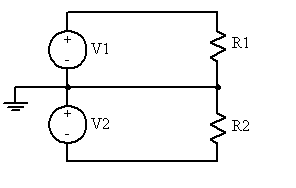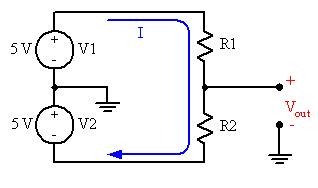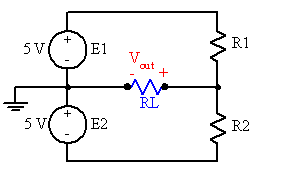# Circuit Analysis

• Engineering
ckboii89

## Homework Statement

Picture of circuit is posted in the Screen Shot.1. Analysis: Both batteries are 5V. Find the values of R1 and R2 that will give an output voltage of 2.5, and a supply current of 1 mA. Then find the values that will give the correct output voltage with a supply current of 5 mA.

## Homework Equations

Ohm's Law
Voltage Divider

Vbatteries(Vbattery1 + Vbattery2) - V1 - V2 = 0 (KVL), I may be wrong, I'm not sure if Vout is part of the equation?

V = IR
V1 = I*R
V2 = I*R

V1 = V * R1/R1+R2
V2 = V * R2/R1+R2

## The Attempt at a Solution

I have not attempted yet, but rather my question is big V(t) the combination of both batteries?
Is Vout taken into account for KVL (aka ground?)
This is a pre-lab question and the professor's notes have not gone over a situation like this.

#### Attachments

•Screen Shot 2013-10-07 at 4.05.57 PM.jpg
44.5 KB · Views: 575

Mentor
There's something fishy with your circuit as given. The placement of the ground connections means that V1 will be directly connected across R1, and V2 directly across R2. So there's no choice for the potential drops across those resistors, they will be independent of the resistor values. To see this, simply replace the two ground connections with a wire joining the points where they connect to the circuit:You need to spell out where Current 1 and Current 2 are exactly, and where Vout is to be measured.

I think you need to verify that the circuit you've shown is correct in all particulars, as it doesn't seem that it can achieve your requirements in its given form.

#### Attachments

Gold Member
2021 Award
I agree completely w/ gneill's assessment. It's exactly what I was going to say, but he beat me to it.

Mentor
Yeah, the right hand ground symbol is probably supposed to be a Vout node symbol...

iRaid
I was going to post earlier that it seemed like that ground wasn't correct, but I didn't want to look like an idiot LOL

Mentor
I think that Berkeman has the right of it and that the junction of the resistors is in fact the output node, and is referred to ground. I'll offer up this interpretation of the circuit and then wait for the OP to get back to us:#### Attachments

ckboii89
hey guys, sorry for the late response was in class.

@gneill, the circuit you just drew up is the correct one! Sorry for the confusion guys! My fault not drawing the ground and Vout node symbol properly

Mentor
@gneill, the circuit you just drew up is the correct one! Sorry for the confusion guys! My fault not drawing the ground and Vout node symbol properly
No problem. Now that we know what the circuit looks like your questions become much easier to interpretFirst, I think it would be worthwhile straightening out the naming of parameters for your circuit. Your circuit diagram labelled the sources V1 and V2, but you've referred to Vbattery1 and Vbattery2 AND V1 AND V2 in the same equation. So I'm going to assume that V1 and V2 are meant to refer to the potentials across resistors R1 and R2 respectively. "Vbattery1" and "Vbattery2" are a bit unwieldy for pushing about in equations, so how about we call them E1 and E2 respectively?

Yes, your "Big V" is the sum of E1 and E2. If you look at the circuit, when no load is connected at Vout there is only one complete path for current to flow. It traverses both batteries and both resistors, and that's it; There's no current flowing through the ground connections. So your KVL equation is fine for the circuit without load:

letting V = E1 + E2

then: V - I*R1 - I*R2 = 0

Vout is just a label for a particular potential difference on the diagram. It's a measurement point rather than a component, so it doesn't affect the circuit operation and doesn't enter into any of the "active" parts of the circuit equations.

-- HOWEVER --

Beware that if a load is connected at Vout then a new circuit path is created between the Vout node and ground and current will flow out of the Vout node and through the ground connections.

I don't know the details of your upcoming lab so I don't know if connecting loads at Vout is a scenario that will come up. But if it does, you'll want to keep E1 and E2 as separate entities in your KVL as they will appear separately in equations for the loops. Here's another arrangement of your circuit that shows what I mean. It's the same circuit as before only with a load RL connected between Vout and ground. I've made the current path explicit with a wire:For your original circuit without a load, your voltage divider equations will work nicely to determine V1 and V2 if you happen to know R1 and R2. But it looks like you're going to be looking for R1 and R2 given particular Vout and total current.